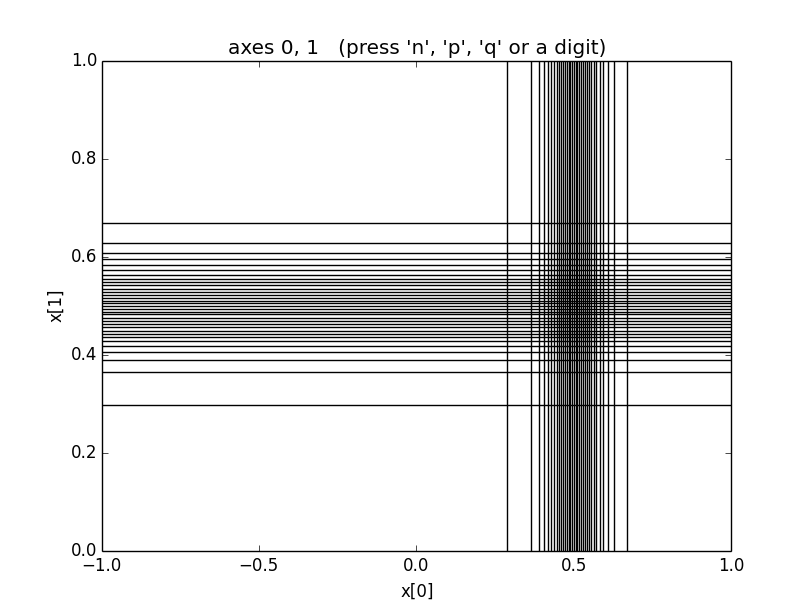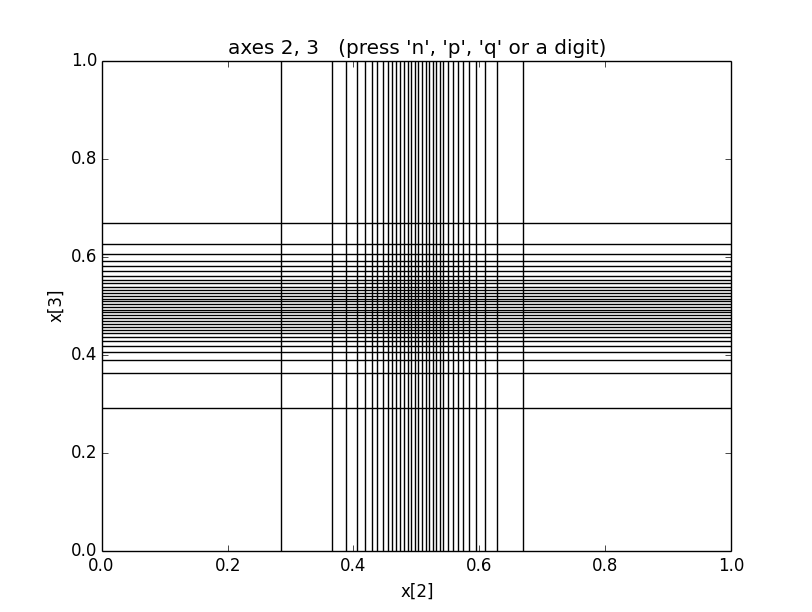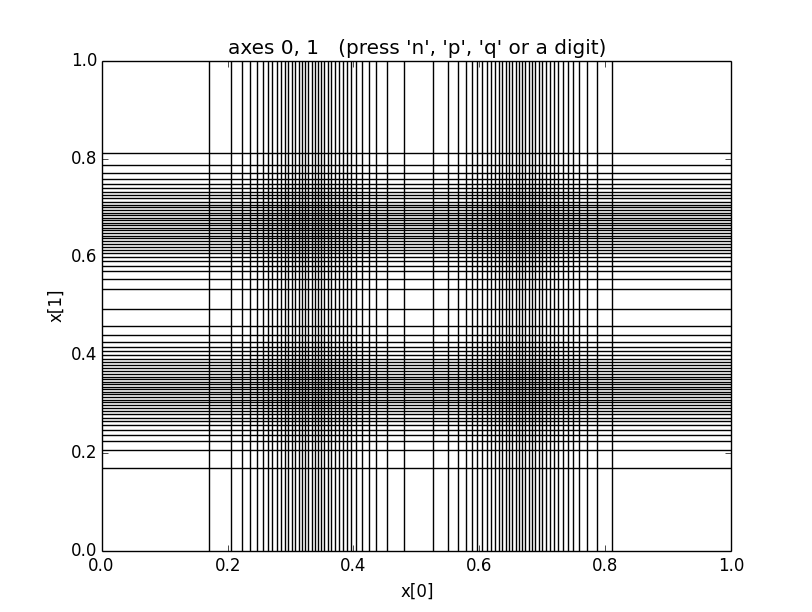# How vegas Works¶

vegas uses two adaptive strategies: importance sampling, and adaptive stratified sampling. Here we discuss the ideas behind each, in turn.

## Importance Sampling¶

The most important adaptive strategy vegas uses is its remapping of the integration variables in each direction, before it makes Monte Carlo estimates of the integral. This is equivalent to a standard Monte Carlo optimization called “importance sampling.”

vegas chooses transformations for each integration variable that minimize the statistical errors in Monte Carlo estimates whose integrand samples are uniformly distributed in the new variables. The idea in one-dimension, for example, is to replace the original integral over,by an equivalent integral over a new variable,whereis the Jacobian of the transformation. A simple Monte Carlo estimate of the transformed integral is given bywhere the sum is overrandom points uniformly distributed between 0 and 1.

The estimateis a itself a random number from a distribution whose mean is the exact integral and whose variance is:The standard deviationis an estimate of the possible error in the Monte Carlo estimate. A straightforward variational calculation, constrained byshows thatis minimized ifSuch transformations greatly reduce the standard deviation when the integrand has high peaks. Sincethe regions inspace whereis large are stretched out inspace. Consequently, a uniform Monte Carlo inspace places more samples in the peak regions than it would if were we integrating inspace — its samples are concentrated in the most important regions, which is why this is called “importance sampling.” The producthas no peaks when the transformation is optimal.

The distribution of the Monte Carlo estimatesbecomes Gaussian in the limit of large. Non-Gaussian corrections vanish like. For example, it is easy to show thatThis moment would equal, which falls like, if the distribution was Gaussian. The corrections to the Gaussian result fall asand so become negligible at large. These results assume thatis integrable for all, which need not be the case ifhas (integrable) singularities.

## The vegas Map¶

vegas implements the transformation of an integration variableinto a new variableusing a grid inspace:The grid specifies the transformation function at the pointsfor:Linear interpolation is used between those points. The Jacobian for this transformation function is piecewise constant:for.

The variance for a Monte Carlo estimate using this transformation becomesTreating theas independent variables, with the constraintit is trivial to show that the standard deviation is minimized whenfor all.

vegas adjusts the grid until this last condition is satisfied. As a result grid incrementsare small in regions whereis large. vegas typically has no knowledge of the integrand initially, and so starts with a uniformgrid. As it samples the integrand it also estimates the integralsand use this information to refine its choice ofs, bringing them closer to their optimal values, for use in subsequent iterations. The grid usually converges, after several iterations, to the optimal grid.

This analysis generalizes easily to multi-dimensional integrals. vegas applies a similar transformation in each direction, and the grid increments along an axis are made smaller in regions where the projection of the integral onto that axis is larger. For example, the optimal grid for the four-dimensional Gaussian integral in the section on Basic Integrals looks like:These grids transform into uniformly-spaced grids inspace. Consequently a uniform,-space Monte Carlo places the same number of integrand evaluations, on average, in every rectangle of these pictures. (The average number is typically much less one in higher dimensions.) Integrand evaluations are concentrated in regions where the-space rectangles are small (and therefore numerous) — here in the vicinity of x = [0.5, 0.5, 0.5, 0.5], where the peak is.

These plots were obtained by including the line

integ.map.show_grid(30)


in the integration code after the integration is finished. It causes matplotlib (if it is installed) to create images showing the locations of 30 nodes of the grid in each direction. (The grid uses 99 nodes in all on each axis, but that is too many to display at low resolution.)

A limitation of vegas’s remapping strategy becomes obvious if we look at the grid for the following integral, which has two Gaussians arranged along the diagonal of the hypercube:

import vegas
import math

def f2(x):
dx2 = 0
for d in range(4):
dx2 += (x[d] - 1/3.) ** 2
ans = math.exp(-dx2 * 100.) * 1013.2167575422921535
dx2 = 0
for d in range(4):
dx2 += (x[d] - 2/3.) ** 2
ans += math.exp(-dx2 * 100.) * 1013.2167575422921535
return ans / 2.

integ = vegas.Integrator(4 * [[0, 1]])

integ(f2, nitn=10, neval=4e4)
result = integ(f2, nitn=30, neval=4e4)
print('result = %s    Q = %.2f' % (result, result.Q))

integ.map.show_grid(70)


This code gives the following grid, now showing 70 nodes in each direction:The grid shows that vegas is concentrating on the regions around x=[0.33, 0.33, 0.33, 0.33] and x=[0.67, 0.67, 0.67, 0.67], where the peaks are. Unfortunately it is also concentrating on regions around points like x=[0.67, 0.33, 0.33, 0.33] where the integrand is very close to zero. There are 14 such phantom peaks that vegas’s new integration variables emphasize, in addition to the 2 regions where the integrand actually is large. This grid gives much better results than using a uniform grid, but it obviously wastes integration resources. The waste occurs because vegas remaps the integration variables in each direction separately. Projected on the x axis, for example, this integrand appears to have two peaks and so vegas will focus on both regions of x, independently of what it does along the x axis.

vegas uses axis-oriented remappings because other alternatives are much more complicated and expensive; and vegas’s principal adaptive strategy has proven very effective in many realistic applications.

An axis-oriented strategy will always have difficulty adapting to structures that lie along diagonals of the integration volume. To address such problems, the new version of vegas introduces a second adaptive strategy, based upon another standard Monte Carlo technique called “stratified sampling.” vegas divides the-dimensional-space volume intohypercubes using a uniform-space grid withorstratifications on each axis. It estimates the integral by doing a separate Monte Carlo integration in each of the hypercubes, and adding the results together to provide an estimate for the integral over the entire integration region. Typically this-space grid is much coarser than the-space grid used to remap the integration variables. This is because vegas needs at least two integrand evaluations in each-space hypercube, and so must keep the number of hypercubessmaller than neval/2. This can restrictseverely whenis large.

Older versions of vegas also divide-space into hypercubes and do Monte Carlo estimates in the separate hypercubes. These versions, however, use the same number of integrand evaluations in each hypercube. In the new version, vegas adjusts the number of evaluations used in a hypercube in proportion to the standard deviation of the integrand estimates (inspace) from that hypercube. It uses information about the hypercube’s standard deviation in one iteration to set the number of evaluations for that hypercube in the next iteration. In this way it concentrates integrand evaluations where the potential statistical errors are largest.

In the two-Gaussian example above, for example, the new vegas shifts integration evaluations away from the phantom peaks, into the regions occupied by the real peaks since this is where all the error comes from. This improves vegas’s ability to estimate the contributions from the real peaks and reduces statistical errors, provided neval is large enough to permit a large number (more than 2 or 3)of stratifications on each axis. With neval=4e4, statistical errors for the two-Gaussian integral are reduced by more than a factor of 3 relative to what older versions of vegas give. This is a relatively easy integral; the difference can be much larger for more difficult (and realistic) integrals.